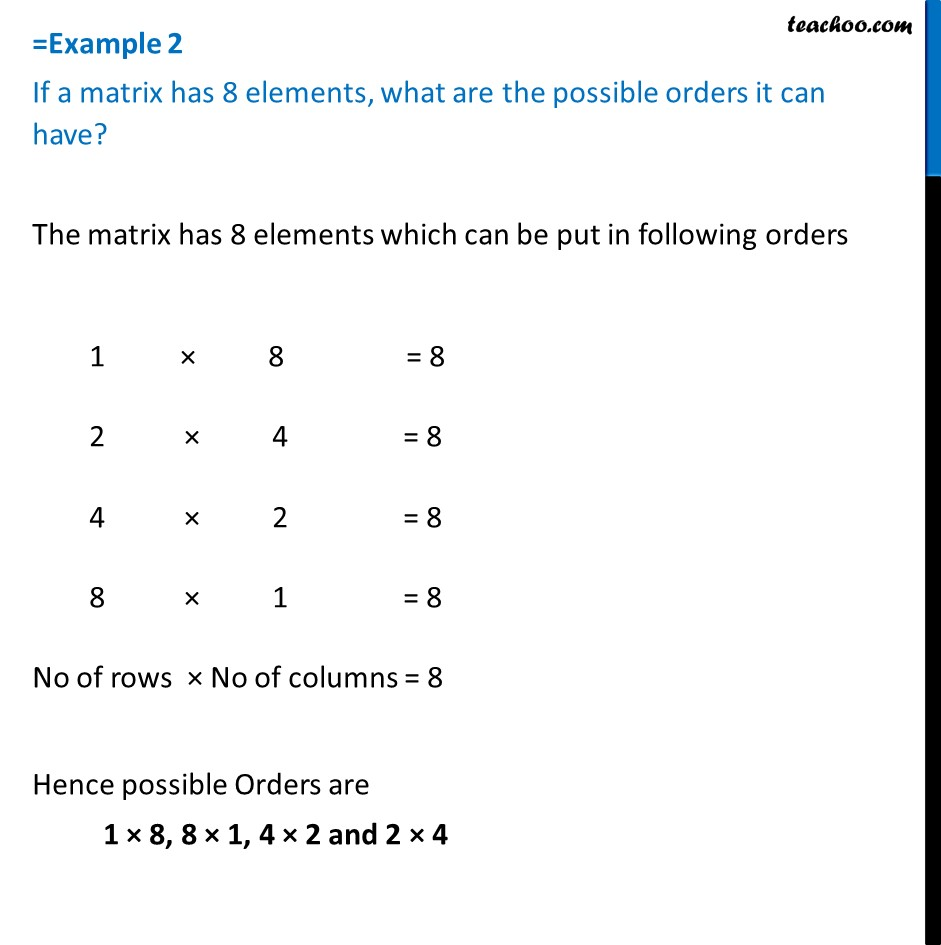Examples

Chapter 3 Class 12 Matrices
Serial order wiseLearn in your speed, with individual attention - Teachoo Maths 1-on-1 Class

### Transcript

=Example 2 If a matrix has 8 elements, what are the possible orders it can have? The matrix has 8 elements which can be put in following orders 1 × 8 = 8 2 × 4 = 8 4 × 2 = 8 8 × 1 = 8 No of rows × No of columns = 8 Hence possible Orders are 1 × 8, 8 × 1, 4 × 2 and 2 × 4